## PHYS 320 Analytical Mechanics

Fall Semester 2018

Dr. Gregory W. Clark
Department of Physics
Office: SCIC 112, Phone: 260.982.5071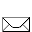Email me!

Here's the syllabus, in case you need another one! Also, here is the Lab Grading Rubric.

You can find .pdf files of the class PowerPoints HERE.

Here are some basics of maple. Here's a Maple worksheet on Maple Position Vectors.

More info on the Research Papers.  CRITIQUE SHEETS are here.

Information on Academic Dishonesty and Grievance.

General Physics Knowsheets: PHYS210 Knowsheet.f18 phys220 knowsheet.s18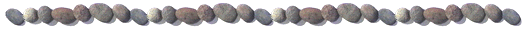Homework assignments:

* HW 25 - Not collected, but work these out before the exam. Solutions are in the binder soon.

• Read all Taylor sections we've discussed this semester (well, at least the bits that are still unclear)!
• Do Taylor problems 7.15, 7.16, 7.17

* HW 24 - Not collected, but work these out before the exam. Solutions are in the binder.

• Read Taylor sections 7.5 - 7.8, 13.1 - 13.3 + 11.4
• Do Taylor problems 7.29, 7.34, 7.35

* HW 23 - Due Tuesday, 04 Dec 2018.

• Read Taylor sections 7.5 - 7.8, 13.1 - 13.3
• Do Taylor problems 7.27, 7.36, 7.38 (a & b), 7.41

* HW 22 - Due Friday, 30 Nov 2018.

• Read Taylor sections 7.3, 7.5
• Do Taylor problems 7.10, 7.17, 7.23, 7.31

* HW 21 - Due Tuesday, 27 Nov 2018.

• Read Taylor sections 7.1 - 7.2
• Do Taylor problems 7.1, 7.2, 7.3
• AQI: Use geometric arguments to derive expressions for the cylindrical coordinate unit vectors r hat and phi hat in terms of the Cartesian unit vectors. Don't worry about k hat, because it's the same in both systems. [HINT: Draw these two vectors in the first quadrant of an x-y plane and find the components.]

* HW 20 - Due Tuesday, 20 Nov 2018.

• Read Taylor sections 7.1 - 7.2
• Do Taylor problems 6.9, 6.10, 6.11

* HW 19 - Due Friday, 16 Nov 2018.

• Read Taylor sections 7.1 - 7.2
• Do Taylor problems 6.4, 6.8, 6.12

* HW 18 - Due Tuesday, 13 Nov 2018

* HW 17 - Due Friday, 09 Nov 2018

* HW 16 - Not collected, but shoot for having these completed before the exam. Partial solutions are in the binder.
• Read Taylor sections 5.6 - 5.8
• Do Taylor Problems: 5.33, 5.36, 5.37
• Additional questions:
• AQ I: Prove mathematically that the maximum in the expression for the amplitude of a driven damped oscillator is given by equation (5.73) in Taylor's text.
• AQII: Insure that you can derive Taylor equations (5.64) and (5.65) by assuming a particular solution to (5.45) of x(t) = A exp(ω t - δ), as we did in class on 30 Oct 2018.
* HW 15 - Not collected, but shoot for having these completed by Tuesday, 30 Oct 2018. Solutions are in the binder.
• Read Taylor sections 5.6 - 5.8
• Do Taylor Problems: 4.36, 5.25, 5.26, 5.29, 5.30
• Additional questions:
• AQ I: Suppose the potential energy of a system is given by U(x) = Uo (4x3 + 2x4).
A. Use Maple to plot the potential.
B. Find the minimum value of the potential (analytically).
C. Find the frequency for small oscillations about this point.
D. Find the force corresponding to this potential. Numerically integrate the differential equation that results from the application of Newton's second law. Concentrate on the equilibrium point. Plot the motion as a function of time.
• AQII: Use the expression for finding the frequency of small oscillations that we developed in class (based on the Taylor series expansion of the potential energy) to derive an expression for the frequency of small oscillations for a simple pendulum. As a starting point, use the expression that you worked with in problem 4.34 for the potential energy. Be careful when you take the derivative; it must be with respect to position, not angle. Write the position, s, in terms of the arclength of the circle along which the pendulum moves (s = L θ) and take the differential of that expression.
* HW 14 - Due Friday, 26 Oct 2018
• Read Taylor sections 5.6 - 5.8, 4.1 - 4.8
• Do Taylor Problems: 4.12, 4.16, 4.20, 4.21, 4.23, 4.34, 5.22, 5.32
• Additional questions:
• AQ I: For the overdamped oscillator, use Maple to produce a phase diagram for each of the three possible initial conditions for an initial positive displacement from equilibrium of xo: (a) vo > 0, (b) vo = 0, and (c) vo < 0. Submit your Maple files to me via email using this file naming format: PHYS320HW14_AQI_XYZ.mw, where XYZ = your initials.

* HW 13 - DueTuesday, 23 Oct 2018

* HW 12 - Due Friday, 19 Oct 2018
• Read Taylor sections 5.4 - 5.5
• Do Taylor problems 5.1, 5.8, 5.11
• Additional questions:
• AQ I: Use Maple to plot v(t) vs. x(t) for the simple harmonic oscillator for one complete cycle of motion. This will be a parametric plot.
• AQ II: A body of uniform cross-sectional area A and mass density D floats in a liquid of density Do and at equilibrium displaces a volume of V. Find an expression for the frequency for small oscillations about the equilibrium position. [You will need to look up Archimedes’ principal for this one; the so-called “buoyant force” plays a critical role]
• AQIII: Two springs have spring constants k1 and k2, and are used in a vertical position to support a single object of mass m. Show that the angular frequency of oscillation is [(k1 + k2)/m]1/2 if the springs are tied in parallel, and [k1k2/(k1 + k2)m]1/2 if they are tied in series. [I.e., find the effective spring constant in each case. ]
* HW 11 - Due Tuesday, 16 Oct 2018
• Read Taylor sections 4.3 - 4.7, 5.1 - 5.3
• Do Taylor problems 2.53, 3.1, 3.3 (these two will help serve as a brief review of the law of conservation of linear momentum from Gen Phys I), 5.5, 5.6
• Additional questions:
• AQ I: Which of the following pairs of functions are linearly independent?
• (A) f1 = sin2(x) and f2 = 1 - cos(2x)
• (B) g1 = e2x and g2 = sinh(x)
• AQ II: A particle undergoes simple harmonic motion with a frequency of 10 Hz. Find the displacement x(t) for any time t for the following initial conditions: x(0) = 0.25 m and v(0) = 0.1 m/s.

* HW 10 - Due Friday, 12 Oct 2018

• Read Taylor sections 2.5 - 2.7, 4.1 - 4.2, 5.1 - 5.2
• Do Taylor problems 2.2, 2.3, 2.45, 2.46, 2.47
• NOTE: For all Maple coding, be sure to include explanatory notes within your Maple file. Submit your Maple files to me via email using this file naming format: PHYS320HW10_AQI_XYZ.mw for AQI. Here, XYZ = your initials.
• AQI: Maple Problem: A parachutist of mass 70 kg jumps from a plane at an altitude of 32 km above the surface of the Earth. Unfortunately, the parachute fails to open. :( Assume the initial velocity is zero and neglect any horizontal motion. (A) Calculate the time of fall (accurate to 1 s) until ground impact assuming constant g and a quadratic air resistance force F(v) = -c v |v| where c = 0.5 in SI units for a falling human. (B) Calculate the time of fall (accurate to 1 s) until ground impact, given that the constant c scales with atmospheric density: c = 0.5 e-y/H where H = 8 km is the scale height of the atmosphere and y is the height above the ground. In addition, assume that g is not constant, but varies according to Newton's Law of Gravity: g = (9.8 m/s2)/(1 + y/RE)2 where RE is the radius of the Earth (6370 km). For each case, plot velocity and altitude of the parachutist as a function of time. Discuss the acceleration (by looking at the v(t) plot) - why does it change direction?!

* HW 9 - Due Friday, 05 Oct 2018

• Read Taylor sections 2.3 - 2.4
• Do Taylor problems 2.20 [use Maple], 2.23, 2.28, 2.33 (a-d)
• NOTE: For all Maple coding, be sure to include explanatory notes within your Maple file. Submit your Maple files to me via email using this file naming format: PHYS320HW09_P2.20_XYZ.mw, for example, for problems from Taylor like problem 2.20 and PHYS320HW09_AQII_XYZ.mw, for example, for AQII. Here, XYZ = your initials.
• AQI: Find the forces in each member of the truss below. State whether each member is in tension or compression.
•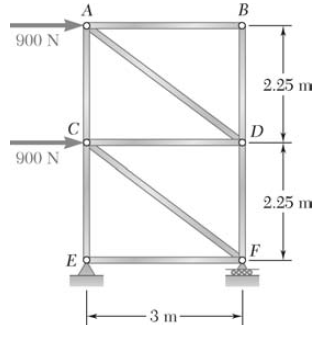• AQII. Maple Problem: Plot v(t) for a dropped object with (A) linear drag and (B) quadratic drag on the same graph [choose the drag coefficients such that both cases have the same terminal velocity]. (C) Next plot v(t) for an object that is thrown downward with an initial speed greater than the terminal speed for each case.

* HW 8 - Not collected! Solutions in binder.

• Read Taylor sections 2.3 - 2.4 [For Tuesday, 02 Oct 2018]
• Do Taylor problems 2.10, 2.11

* HW 7 - Due Tuesday, 25 Sept 2018

* HW 6 - Due Friday, 21 Sept 2018

• Read Taylor sections 2.1 - 2.2
• Do Taylor problems 2.9, 2.14
• Do additional questions:
• AQI: Find the velocity, dx/dt, as a function of the displacement, x, for a particle of mass m, which begins from rest at x = 0 and t = 0, subject to the following forces: (a) Fx = Fo + c x, (b) Fx = Fo cos(cx), and (c) Fx = Foe -cx where Foand c are positive constants. Hint: Use the second law and separation of variables!
• AQII. The force acting on a particle of mass m is given by F(x,v) = k v x, where k is a positive constant. The particle passes through the origin with a speed vo at a time t = 0. Find x as a function of t.
• AQIII: A uniform horizontal beam (of weight W = 200N and length L = 8 m) is attached to a wall via a hinge. The far end is supported by a cable that makes an angle of 53 degrees relative to the horizontal. If a 600 N person stands 2 m from the hinge, find the tension, T, in the cable and the reaction force at the hinge (force exerted by the hinge on the beam).
•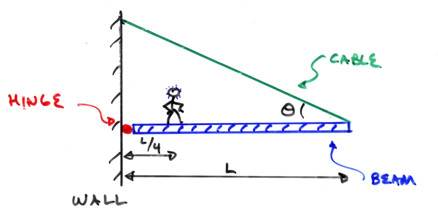• AQIV: A uniform ladder of length L and weight W = 50 N rests against a smooth vertical wall. If the coefficient of static friction is 0.40, find the minimum angle (theta, in the diagram below) such that the ladder will not slip.
•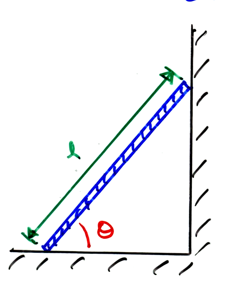* HW 5 - Due Tuesday, 18 Sept 2018

• Read Taylor sections 2.1 - 2.2
• Do Taylor problems 1.36, 1.39, 2.7, 2.8, 2.12, 2.13

* HW 4 - Due Friday, 14 Sept 2018

• Read Taylor sections 1.5 - 1.7, 2.1 - 2.2
• Do Taylor problems 1.26, 1.35, 1.37
• Do additional questions:
• I. (a) If you are sitting quietly at a table, what is your speed as you move around the Earth's rotation axis {the latitude at North manchester is 41 degrees north; it takes 23 hours and 56 minutes for the Earth to revolve once around in a circle on its axis.)? What is your acceleration due to this motion of the Earth spinning on its axis? What percentage of the free-fall acceleration is this?
• I (b) If you are sitting quietly at a table, what is your speed as you move in your orbit around the Sun? What is your acceleration due to this orbital motion around the Sun? What percentage of the free-fall acceleration is this? [Ignore the spin of the Earth on its axis. The Earth's orbit around the Sun is elliptical, but the ellipse is fairly close to being a circle; approximate the orbit as a circle using the mean orbital radius.]
• I. (c) Considering both orbital and rotational motion, at about what time of day are you moving the fastest relative to the Sun? The slowest? Calculate both speeds.
• II. Find the velocity, dx/dt, and the position, x, as functions of the time, t, for a particle of mass m, which begins from rest at x = 0 and t = 0, subject to the following forces: (a) Fx = Fo + c t, (b) Fx = Fo sin(ct), and (c) Fx = Foe c t where Foand c are positive constants. Hint: Use the second law and separation of variables!

* HW 3 - Due Tuesday, 13 Sept 2018

• Read Taylor sections 1.5 - 1.7, 2.1 - 2.2
• Do Taylor problems 1.11, 1.19, 1.24, 1.25
• Do additional questions:
• I. A small ball is attached to a long rubber band and twirled around so that the ball moves in a path given by r(t) = i b cos(ωt)+j 2 b sin(ωt) where b and ω are constants. What is the shape of this path (trajectory)? Find the speed, v, of the ball as a function of t. Next, find the values of v at t = 0 and t =π/2ω , at which times the ball is, respectively, at its minimum and maximum distances from the origin.
• II. The position of a fly as a function of time is given by r(t) = i (1 - e-kt) + j ekt where k is a positive constant. Find the velocity and acceleration of the fly and sketch its trajectory.
• III. A wheel of radius b rolls along the ground with constant forward acceleration ao. Show that, at any given instant, the magnitude of the acceleration of any point on the wheel is ( ao2 + v4 / b2)1/2 relative to the center of the wheel and is ao[ 2 + 2 cos θ + v4 / (ao2b2) - (2 v2 / (aob)) sin θ ]1/2 relative to the ground. Here v is the instantaneous forward speed and θ gives the location of the point on the wheel in question, measured forward from the highest point (i.e., relative to the vertical, as we used in class). Which point has the greatest acceleration relative to the ground?
• IV. Prove that v·a = v (dv/dt) and, hence, that for a moving particle v and a are perpendicular to other if the speed v is constant. (Hint: Differentiate both sides of v·v = v2 with respect to t. Note that dv/dt is NOT the same as |a|! It is the magnitude of the acceleration of the particle along its instantaneous direction of motion.) [NOTE: Here, bold font indicates a vector!]

* HW 2 - Due Friday, 7 Sept 2018
• Read Taylor sections 1.3 - 1.7
• Do Taylor problems 1.9, 1.10, 1.12
• Do additional questions:
• I. Use the definition of the cross product C = A x B thatto show, by explicitly carrying out the two sums, that you get the expected result for C2 (= Cy).
• II. Prove the "BAC-CAB" rule by either brute force (i.e., pluggin in three completely general vectors) or using the Levi-Civita symbol:
•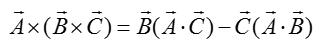• I II. One of my friends has chosen a life of crime.  He has become a bird smuggler.  He smuggles birds over the border via a closed back truck (basically, a closed box).  He’s got this great idea for foiling the customs officers at the border, where his truck will be weighed on a spring scale.  Just before reaching the scale with his truck full of birds, he will reach out through the window and bang on the back of the truck really hard in order to scare the birds into flight (hovering).  Since they will no longer be sitting on the floor of the truck, the truck will weigh its normal, unloaded weight.  The customs folks will, thus, assume the truck is empty and he will get through with no problem.  Will his plan work?  [He’s not concerned about sound because these are rare, purple-breasted Newton-birds, which are, as it happens, voiceless.]

* HW 1 - Due Tuesday, 04 Sept 2018

• Read Taylor sections 1.1 - 1.4
• Do Taylor problems 1.1, 1.2, 1.4, 1.5, 1.6
• Do the review questions in this worksheet: vector product practice.f18
• Do additional question:
I. You've won an all expenses paid vacation to the Bahamas and, en route via airplane, you ask the stewardess for a glass of ginger ale. You are flying at an altitude of 35,000 ft at a constant velocity of 300 mph, southeast. Your attention to the scenery out the window is distracted by the bubbles in your glass as they rise to the surface of the ginger ale. A. Describe the path of the bubbles. B. How does this motion of the bubbles compare to their motion during takeoff (high acceleration)? C. What about during landing (high deceleration)?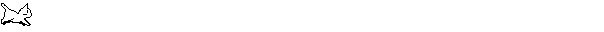Back to my main web page.